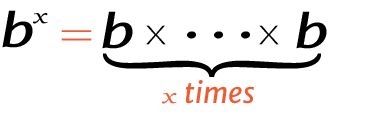# 81 raise to the power of 2

Place the base and exponent in the power calculator to get the right answer for value of 2^81, 281 (2 power 81), or 81 raised to the power of 2.

## How to calculate 2 raise to the power of 81?

This is how you can solve (2) 81 manually.

Solution:

Step 1: Simplify the data.

Base
ExponentStep 2: Multiply the 2 to itself 81 times.

Using this exponents calculator could be much easier to figure out an exponential expression.